Open in App
Not now

# How to Truncate a File in Golang?

• Last Updated : 23 Mar, 2020

In Go language, you are allowed to truncate the size of the file with the help of the Truncate() function. This function is used to truncate the size of the given file in the specified size.

• If the given file is a symbolic link, then it changes the size of the link’s target.
• If this method throws an error, then it will be of type *PathError.
• It is defined under the os package so, you have to import os package in your program for accessing Truncate() function.
• Suppose if the truncate file if of 100 bytes and the original file is less than 100 bytes, then the original content remains at the beginning and the remaining space is filled with the null bytes. And if the original file is greater than 100 bytes, then the truncated file lost content after 100 bytes.
• If you pass 0 in the Truncate() function, then you will get an empty file.

Syntax:

`func Truncate(name string, size int64) error`

Example 1:

 `// Golang program to illustrate how to``// truncate the size of the given file``package main`` ` `import (``    ``"log"``    ``"os"``)`` ` `var (``    ``myfile *os.FileInfo``    ``e      error``)`` ` `func main() {``  ``// Truncate the size of the ``  ``// given file to 200 bytes ``  ``// Using Truncate() function``    ``err := os.Truncate(``"gfg.txt"``, 200)``    ``if` `err != nil {``        ``log``.Fatal(err)`` ` `    ``}``}`

Output:

Before: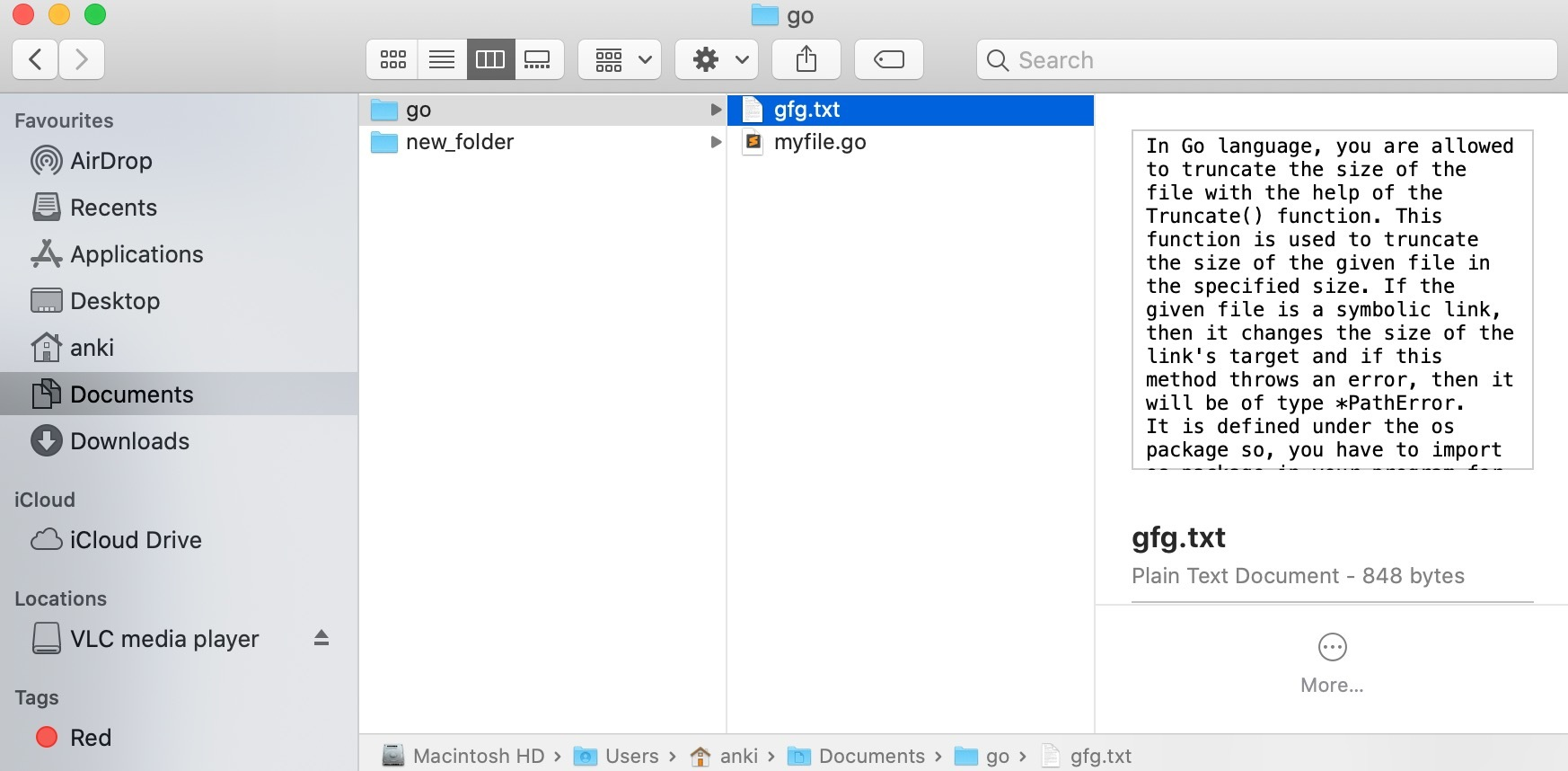After: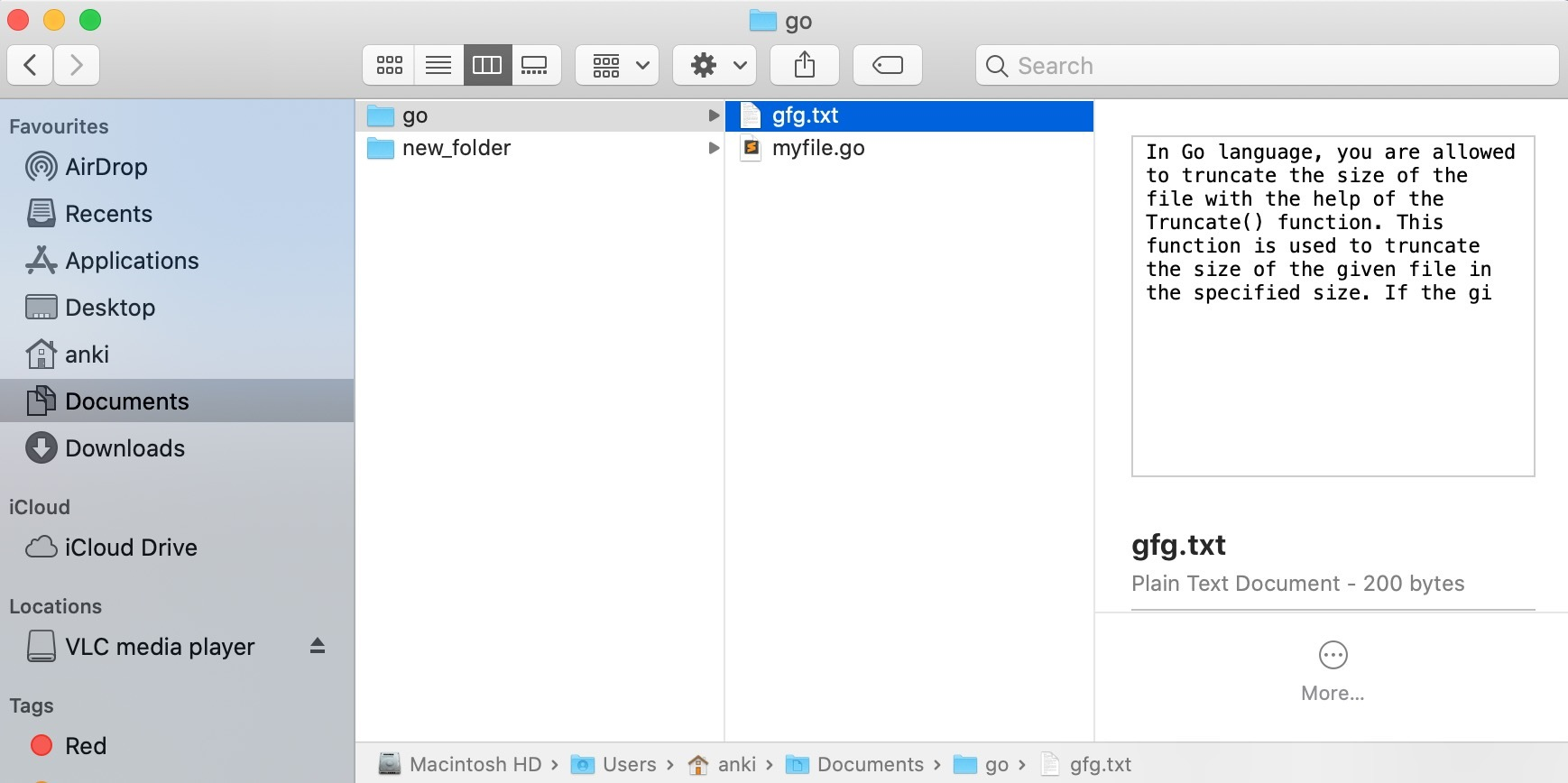Example 2:

 `// Golang program to illustrate how to``// truncate the size of the given file``package main`` ` `import (``    ``"log"``    ``"os"``)`` ` `var (``    ``myfile *os.FileInfo``    ``e      error``)`` ` `func main() {``  ``// Truncate the size of the given ``  ``// file to 0 bytes or empty file``  ``// Using Truncate() function``    ``err := os.Truncate(``"gfg.txt"``,0)``    ``if` `err != nil {``        ``log``.Fatal(err)`` ` `    ``}``}`

Output:

Before:After: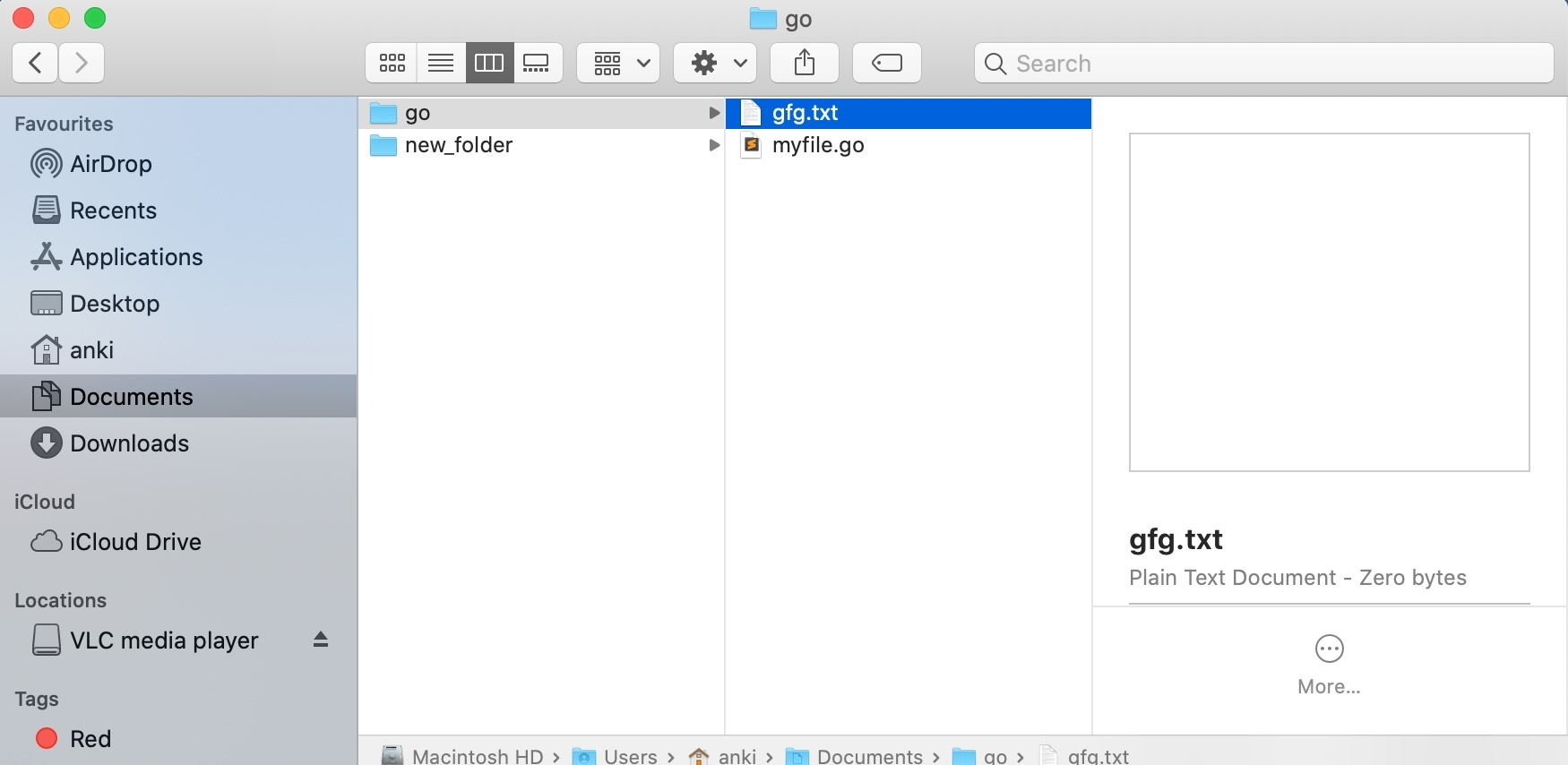Example 3:

 `// Golang program to illustrate how to ``// truncate the size of the given file``package main`` ` `import (``    ``"log"``    ``"os"``)`` ` `var (``    ``myfile *os.FileInfo``    ``e      error``)`` ` `func main() {``  ``// Truncate the size of the ``  ``// given file to 300 bytes ``  ``// Using Truncate() function``    ``err := os.Truncate(``"/Users/anki/Documents/new_folder/bingo.txt"``, 300)``    ``if` `err != nil {``        ``log``.Fatal(err)`` ` `    ``}``}`

Output:

Before: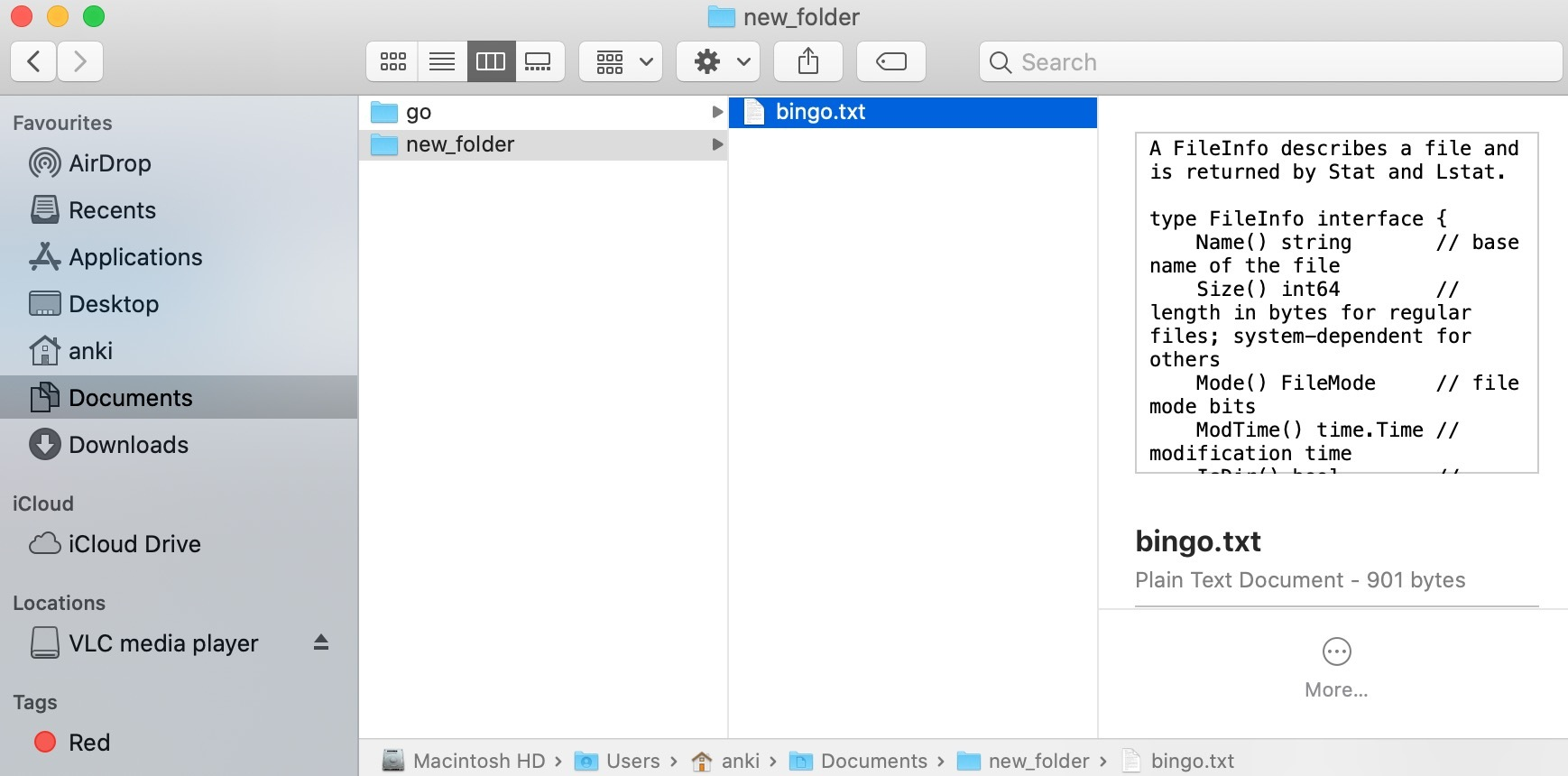After: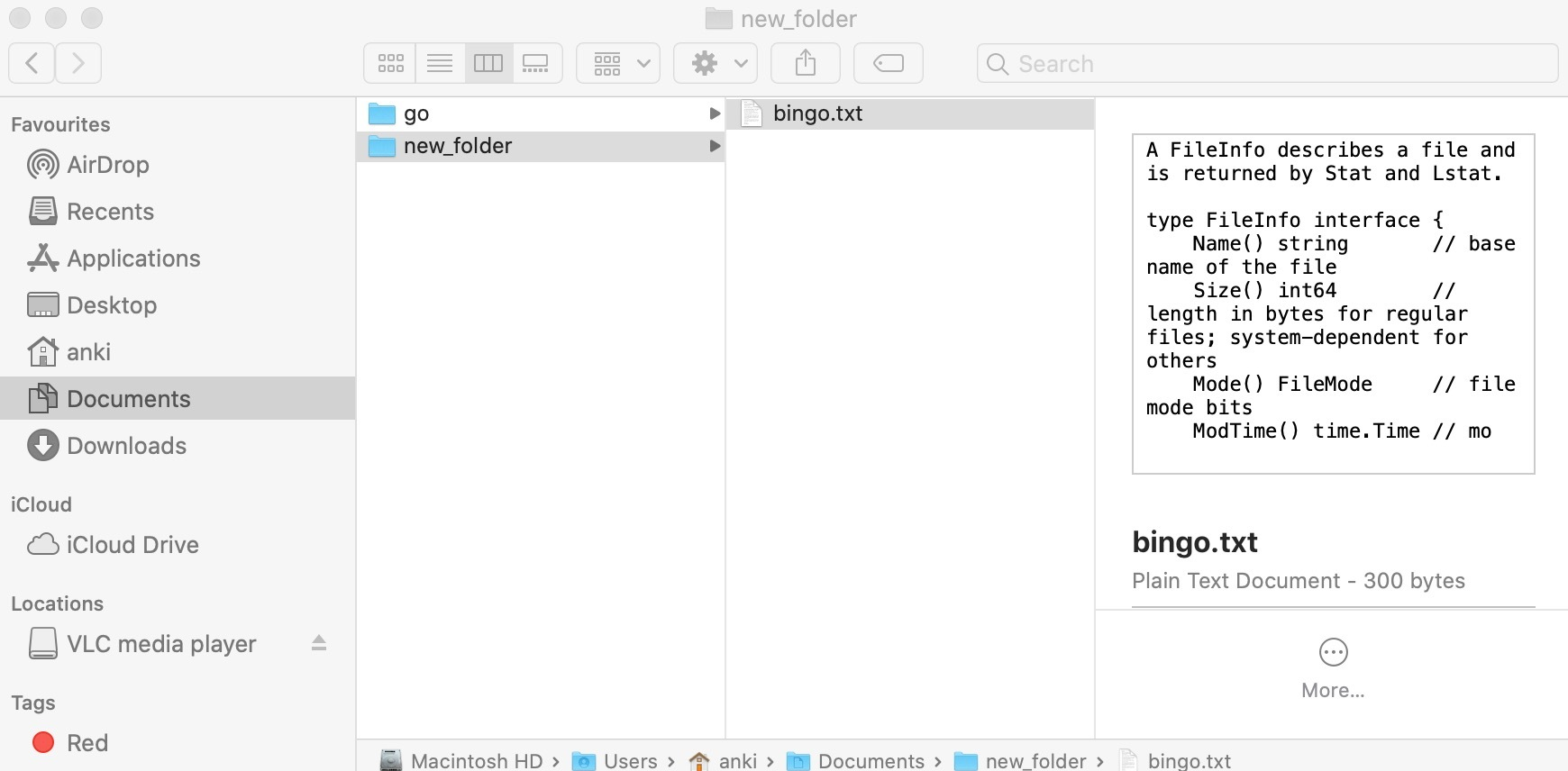My Personal Notes arrow_drop_up